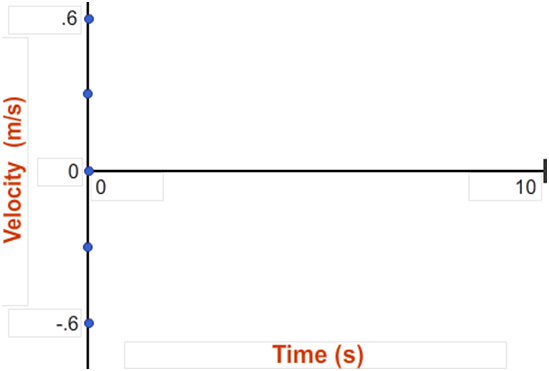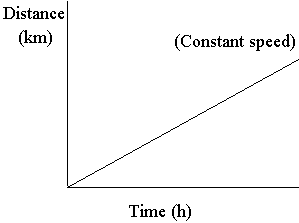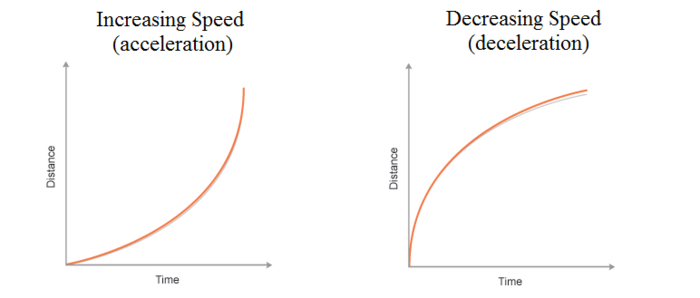# How to convert an x-t graph into a v-t graph?

Dear Student,

Converting a x-t graph to v-t graph comes with multiple rules.

1) IF THE x-t GRAPH IS PARALLEL TO X AXIS:This denotes the velocity of the object is zero (thus it's distance is not changing).2) IF THE x-t GRAPH IS A STRAIGHT DIAGONAL LINE:This denotes that we have a velocity value but it has constant velocity, thus, the corresponding graph will be:

​​​​or3) IF THE x-t GRAPH IS CIRCULAR:This corresponds to diagonal slope in v-t graph.orRegards

• 1
What are you looking for?